Thursday, October 6, 2022

# Arduino Based Digital Capacitance Meter: An Interrupt Based Approach

Saikat Patra is passionate about electronics and MCU based embedded system applications -- Shibendu Mahata is M.Tech (gold medallist) in instrumentation and electronics engineering from Jadavpur University. Currently, he is pursuing Ph.D from NIT, Durgapur. He is interested in MCU based real-time embedded signal processing and process control systems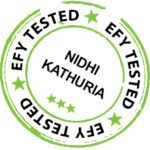Capacitive sensors are widely used to measure various physical and chemical process parameters such as displacement, acceleration, thickness, force, pressure, stress, level and humidity. The measured value of capacitance is then calibrated in terms of the process parameter for indication and/or control. This project presents an interrupt based approach by employing an NE555 timer and Arduino Uno to measure capacitance in the range of 1µF to 1mF, and provide local indication using an LCD and data acquisition using a PC display (serial monitor of Arduino IDE). The authors’ prototype of the digital capacitance meter is shown in Fig. 1.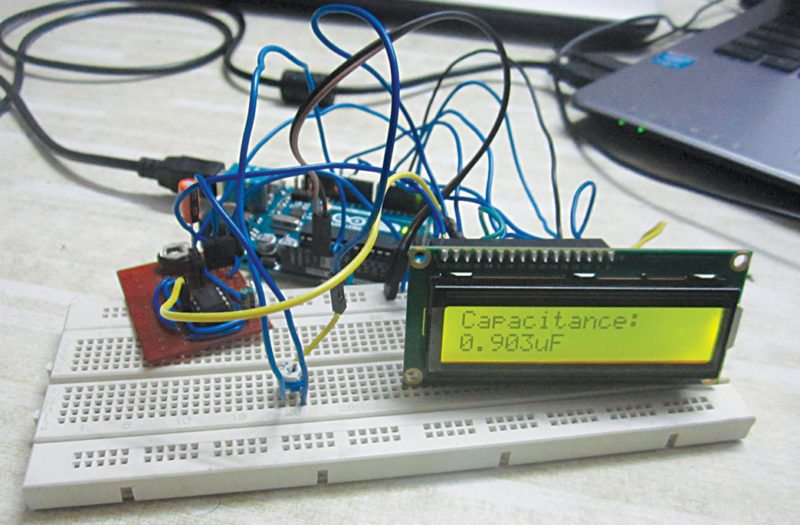Fig. 1: Authors’ prototype of Arduino based digital capacitance meter

Two methods have been described here: first with NE555 timer configured in astable multivibrator mode and second with NE555 timer configured in monostable mode.

# Capacitance meter circuit and working

Block diagram of Arduino based digital capacitance meter is shown in Fig. 2 and its circuit diagram with NE555 timer in astable multivibrator mode is shown in Fig. 3.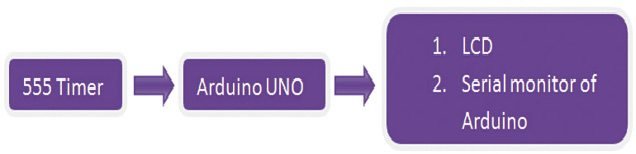Fig. 2: Block diagram of Arduino based digital capacitance meter

Here, NE555 timer (IC1) is operated with two external resistors (R1 and R2) and an unknown capacitor (Cx), whose value is to be measured. IC1 is powered with +5V from Arduino board (Board 1); thus, eliminating the need for an external DC power supply.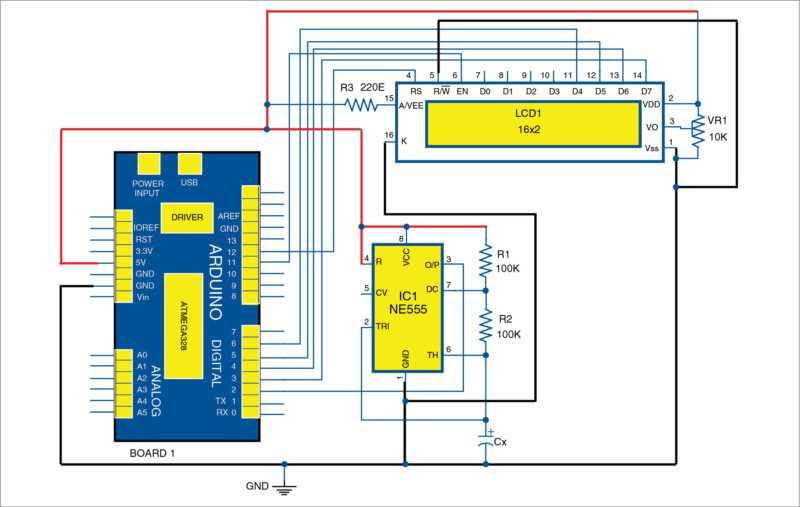Fig. 3: Circuit diagram of Arduino based digital capacitance meter with NE555 timer in astable mode

IC1 generates a square-wave output of +5V amplitude at a specific frequency (depending on values of R1, R2 and Cx), which is available at its output pin 3. Output pin 3 of IC1 is connected to pin 2 of Arduino board. Since this pin of Arduino is a hardware interrupt pin (called Interrupt 0), source code (capacitance1.ino) uploaded to Arduino uses an interrupt handler, which is executed whenever the timer output makes a low-to-high transition. Thus, time period of the square-wave is continuously obtained by calculating the time difference between two such consecutive transitions. Time-period (T) of oscillation for the square-wave output from IC1 is given as:

T=0.693×(R1+2×R2)×Cx

Thus, value of Cx is given as:

Cx=1.443×T/(R1+2×R2)

Measured value of Cx (in µF) is then displayed on a 16×2-character LCD and PC.

## NE555 timer

NE555 timer IC1 operates as an oscillator in astable multivibrator mode with free-running frequency, and duty cycle is accurately controlled by R1, R2 and Cx. Pins 4 and 8 of IC1 are connected to +5V connector of Board 1.

R1 and R2 of 100-kilo-ohm each are connected between pins 6 and 7, and pins 7 and 8, respectively, of IC1. IC1 shares the same ground with Arduino board. The author’s designed breakout board for the timer is shown in Fig. 4.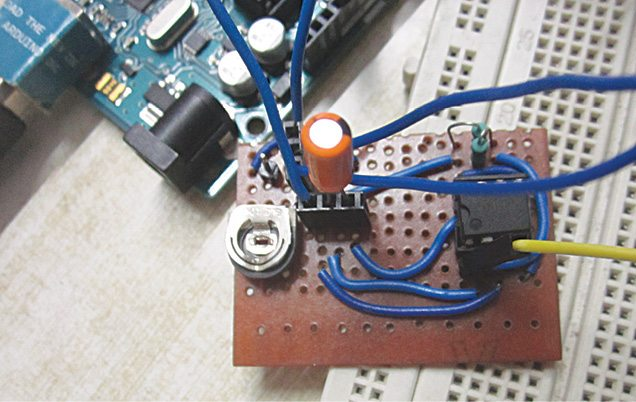Fig. 4: NE555 timer connection in author’s breakout board

## Arduino Uno board

Arduino Uno is an AVR ATmega328 microcontroller based development board with six analogue input pins and 14 digital I/O pins. The microcontroller has 32kB of ISP flash memory, 2kB RAM and 1kB EEPROM. The board provides serial communication via UART, SPI and I2C.

The microcontroller can operate at a clock frequency of 16MHz. In this project, digital I/O pins 3, 4, 5, 6, 11 and 12 of Arduino are connected with pins 14, 13, 12, 11, 6 and 4, respectively, of the LCD.

16×2 character LCD. Since our Arduino program (capacitance.ino) uses the LCD in 4-bit mode, only LCD data lines D3-D7 are configured for reading data from Arduino. Pins 1 and 2 of the LCD are connected to GND and 5V, respectively, from Arduino board. Read/write pin (pin 5) of the LCD is connected to GND. A 10-kilo-ohm potentiometer (VR1) is provided for adjusting the contrast of the display.

# Software

The source code (capacitance1.ino) is written in Arduino programming language. Atmega328/Atmega328P is programmed using Arduino IDE software. Select the correct board from Tools→Board menu in Arduino IDE and burn the program (sketch) through the standard USB port in your computer.

Here, code written in Arduino uses LiquidCrystal.h header file provided by Arduino library for working with the LCD.

lcd.begin(16, 2) function helps configure the 16×2 character LCD.

Serial.begin(9600) function initialises the serial port with a baud rate of 9600.

attachInterrupt(0,cap,RISING) function calls interrupt handler ‘cap’ whenever a signal connected to interrupt 0 pin (pin 2) of Arduino makes a low-to-high, that is, rising-edge transition.

Serial.print(capacitance, 3) function prints the measured value of capacitance up to three decimal places using the serial port on the PC monitor.

lcd.setCursor(0, 1) function sets the LCD cursor position to print from first column of second row.

lcd.print(capacitance, 3) function prints the measured value of capacitance up to three decimal places on the LCD screen.

# NE555 timer in monostable mode

In the second method (Fig. 5), NE555 timer (IC1) is operated in monostable multivibrator mode with external resistor R1 and an unknown capacitor (Cx), whose value is to be measured. IC1 is powered with +5V from Arduino board (Board 1); thus, eliminating the need for an external DC power supply.

1.Sayantan Maiti

Can we measure unpolarised capacitor values using this method???

2.efylab

Yes, you can measure the values of polarised and unpolarised capacitors.

3.Gireesh kumar G

what is the measuring range ….. can i measure any capacitor ??

•Sani Theo

Please read the article. It is clearly mentioned that this circuit can measure capacitance in the range of 1 micro Farad to 1 milli Farad

4.CHARLES musa

i want to do a school project on Arduino based digital capacitance meter with NE555 timer in astable mode, but didn’t understand it

5.Patrick

I have built the circuit, downloaded the code for capacitance 1 and uploaded it to Arduino and run it The lcd displays Capacitance: 0.000uF even with a 10uf capacitor used as Cx. I don’t know how to solve it . it may be the code. I have double checked the circuit Arduino to LCD display and the 555 but nothing wrong. Perhaps you can help?